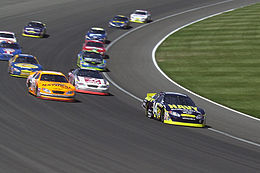Speed, Acceleration, and Velocity
3 years ago
mrsbrockmyre
Save
Edit
Host a game
Live GameLive
Homework
Solo Practice
Practice12 QuestionsShow answers
• Question 1
30 seconds
Q. A child rides a bike around the block.
Speed
Velocity
Acceleration
• Question 2
45 secondsQ. Describe the speed of the rocket in the speed vs. time graph.
Constant speed
Decreasing speed
Increasing speed
Not moving
• Question 3
30 seconds
Q. A dog runs on the sidewalk 9 mi/hr.
Speed
Acceleration
Velocity
• Question 4
30 secondsQ. Describe the speed of the object from 4-6 seconds using the distance vs. time graph.
Increasing speed
Decreasing speed
Not moving
Constant speed
• Question 5
30 seconds
Q. While going to school, a student walks an average of 5 km/hr west for 12 blocks.
Speed
Acceleration
Velocity
• Question 6
30 secondsQ. Describe the speed of the object from
0-2 seconds using the distance vs. time graph.
Constant
Speeding up
Slowing down
Not moving
• Question 7
30 seconds
Q. While on a trip, a family travels east from San Antonio to Houston going 70 mph.
Speed
Acceleration
Velocity
• Question 8
45 secondsQ. Describe the speed of the object from
2-5 seconds using the speed vs. time graph.
Increasing speed
Not moving
Decreasing speed
Constant speed
• Question 9
30 seconds
Q. A bicyclist takes a curved, scenic ride through the park going 4.5 m/s.
Speed
Acceleration
Velocity
• Question 10
45 secondsQ. Describe the speed of the object from
5-6 seconds using the speed vs. time graph
Speeding up
Slowing down
Not moving
Constant speed
• Question 11
30 seconds
Q. Some motorcycles can go from 0 mi/hr to 60 mi/hr in under 4 seconds.